EN | ES | DE | NL | RU

## Solutionns Cheat Sheet

Solutions Cheat Sheet

Solutions are homogeneous mixtures of two or more than two matters. Solutions can be in solid, liquid and gas phases.  Solvent is the medium in which matter is dissolved and solute is the matter that dissolves in solvent. For example, in water sugar solution, water is the solvent that dissolves sugar and sugar is the solute that is dissolved in water.

Dilute Solution: Solutions having small amount of solute in solvent are called dilute solutions.

Concentrated Solution: Solutions in which large amount of solute dissolves in solvent.

Solvation: It is the process of dissolution of solute in solvent.

1) Ionic Solvation: If solvent decomposes into its ions, we call these solutions ionic solutions. Acids, bases and salts produce ionic solutions.

2) Molecular Solvation: If a matter decomposes into its molecules, we call these solutions molecular solutions. Dissolution of sugar in water is example of molecular solvation.

We can examine solutions under two title according to their saturation ratio; such as saturated solutions and unsaturated solutions and supersaturated solutions.

1) Saturated Solutions: If solution dissolves maximum amount of solute at specific temperature, then we call them as saturated solutions. In this type of solutions there can be solid matters (undissolved) at the bottom of tank.

2) Unsaturated Solutions: If solutions can solve more solute at specific temperature, then we cal them unsaturated solutions. If you vaporize some of the solvent or add some solute you can make them saturated solutions.

3) Supersaturated Solutions: If solutions contain more solute than its capacity, we call these solutions supersaturated solutions. We prepare them by heating solution and adding solute, after that we cool slowly supersaturated solution. You can observe crystallization of solute in supersaturated solutions.

Solubility is the amount of solute in 100 cm3 (100 mL) solvent.

Solvent and types of solute, temperature, pressure and common ion effect are factors affecting solubility.

Factors Effecting Speed of Solvation

• Types of matter
• Changing temperature ( decreasing for exothermic solutions and increasing for endothermic solutions)
• Surface of contact (granulated sugar dissolves faster than cube sugar)
• Mixing solution increases speed of solvation.

Concentration is the amount of solute in given solution. We can express concentration in different ways like concentration by percent or by moles.

1) Concentration by Percent:

It is the amount of solute dissolves in 100 g solvent. If concentration of solution is 20 %, we understand that there are 20 g solute in 100 g solution.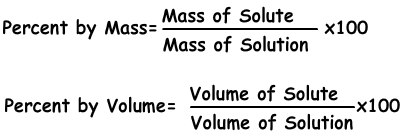2) Concentration by Mole:

We can express concentration of solutions by moles. Number of moles per liter is called molarity shown with M.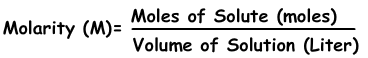3) Molality:

Molality is the another expression of concentration of solutions. It is denoted with "m" and formula of molality is;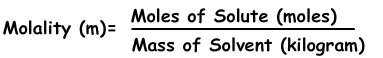4) Normality:

We can express concentration in another way with normality using equivalents of solutes.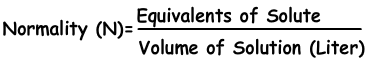Dilution is process of adding solvent to solution. Since amount of solute stays constant, concentration of solution decreases. We find relation between concentration of solutions before and after dilution with following formula;

M1.V1=M2.V2

Where M1 is initial molarity and M2 is final molarity and V1 and V2 are initial and final volumes of solution.

To increase concentration of solutions, you should add solute or evaporate solvent from solution. Formula given above is also used in increasing concentration of solutions;

M1.V1=M2.V2

Density of Solutions

We find density of solutions by following formula;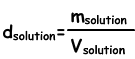• Boiling point, freezing point, vapor pressure, and properties like density of solutions are different from properties of pure solvent. For example, water boils at 100 oC, on the contrary salt water solution boils  above 100 oC.
• If dissolved matter in water is not volatile, they prevent vaporization of water and as a result boiling point of water increases and freezing point of water decreases. In winter, salt is poured on road to decrease freezing point of water.
• In a solution, increasing boiling point is directly proportional to molar concentration of particles in solution.
• In liquid solutions, decreasing of freezing points and vapor pressure is inversely proportional to molar concentration of particles in solution.
• Conductivity of electricity is directly proportional to molar concentrations of ions in solutions. For example, alcohol and sugar do not conduct electricity.

The Original Author: